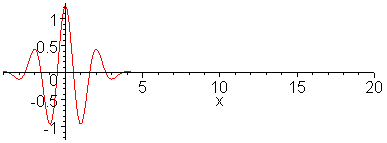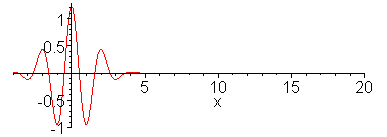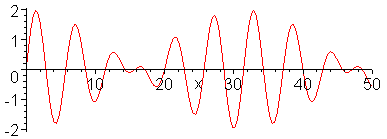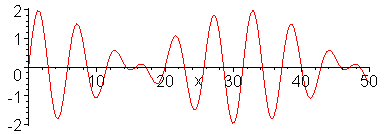### Dispersive Waves

 Dispersive WavesIn a nondispersive wave medium, waves can propagate without deformation Electromagnetic waves in unbounded free space are nondispersive as well as nondissipative and thus can propagate over astronomical distances. Sound waves in air are also nearly nondispersive even in the ultrasonic frequency range. If not, that is, if high frequency notes (e.g., piccolo) and low frequency notes (e.g., base) propagate at different velocities, they would reach our ears at different times, and music played by an orchestra would not be harmonious. Most waves in material media are dispersive, however, and wave forms originally set up are bound to change in a manner that the wave energy is more spatially spread out or dispersed. Animation 1 below shows propagation of dispersive wave packet and Animation 2 nondispersive wavepacket. In making the animation, 100 sinusoidal waves are superposed for a dispersion relation, (The dispersion relation describes the ion acoustic wave in a plasma and also approximately shallow water waves. See, for example, A. Hirose et al., Plasma Physics, Vol. 20, p. 1179 (1978), in which response of the ion acoustic wave to an impulse has been analyzed.) Note that in the dispersive case shown in the top animation, long wavelength components propagate faster than short wavelength components. The envelope of the wave packet propagates at the group velocity. Wave ripples propagate at the phase velocity. In contrast, nondispersive wave packet (Animation 2) described by maintains the original wave form. Animation 3 shows superposition of two sinusoidal waves in dispersive case, sin(x - t) + sin(1.2x - 1.1t) (group velocity = half of phase velocity), while the last animation shows the case of nondisperve wave, sin(x - t) + sin(1.2x - 1.2t). Note that in the dispersive case (Animation 3), wave propagation is not simple parallel shift. 1. Dispersive wavepacket> with(plots): animate(sum(.07*(exp(-(.1*k-3)^2)+exp(-(0.1*k+3)^2))*cos(.1*k*x-.1*k/sqrt(1+.1*(.1*k)^2)*t),k=1..100),x=-4..20,t=0..30,frames=60,numpoints=200,color=red);2. Nondispersive wavepacket> animate(sum(.07*(exp(-(.1*k-3)^2)+exp(-(0.1*k+3)^2))*cos(.1*k*x-.1*k/sqrt(1+.0*(.1*k)^2)*t),k=1..100),x=-4..20,t=0..30,frames=60,numpoints=200,color=red);3. Dispersive sinusoidal waves> animate(sin(x-t)+sin(1.2*x-1.1*t),x=0..50,t=0..63,numpoints=150,frames=100,color=red);4. Nondispersive sinusoidal waves> animate(sin(x-t)+sin(1.2*x-1.2*t),x=0..50,t=0..63,numpoints=150,frames=100,color=red);Ċ
Jason Lin,
2018年9月6日 凌晨12:43